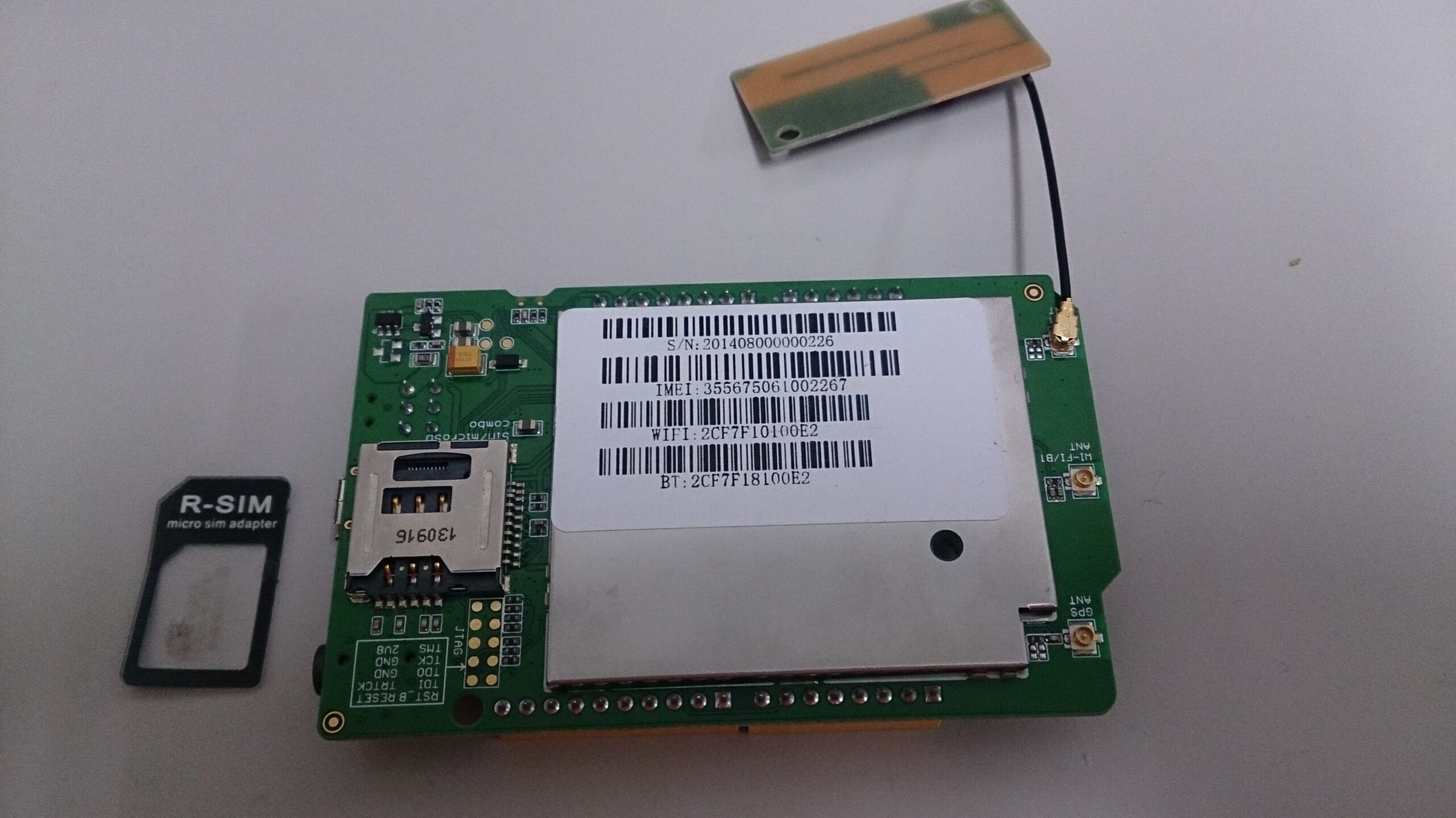``````#include <LTask.h>
#include <LGSM.h>
const int pinLed = 3; // LED接在 D3 腳位

void setup()
{
Serial.begin(9600);
{
delay(1000);
}

Serial.println("GSM OK!!");
}
void loop()
{

char p_num;
int len = 0;
char dtaget;

if(LSMS.available()) // 檢查有沒有新簡訊
{
LSMS.remoteNumber(p_num, 20); //顯示發送者電話號碼
Serial.println("There is new message.");

Serial.print("Number:");
Serial.println(p_num);
Serial.print("Content:"); // 顯示簡訊內容

while(true)
{
if(v < 0)
break;

dtaget[len++] = (char)v;
Serial.print((char)v);
}

Serial.println();
LSMS.flush(); // 刪除簡訊
if((dtaget == 'O' && dtaget == 'N') || (dtaget == 'o' && dtaget == 'n'))
{
//檢查簡訊前兩個字元是否為 ON 或 on
digitalWrite(pinLed, HIGH);
// 這不用多說了吧，亮燈！
}

else if((dtaget == 'O' && dtaget == 'F' && dtaget == 'F') || (dtaget == 'o' && dtaget == 'f' && dtaget == 'f'))
{
digitalWrite(pinLed, LOW);
// 熄燈
}
}
}``````

### 2 thoughts on “[LinkIt One教學] – 簡訊控制LED亮滅”

1.JF says:

•